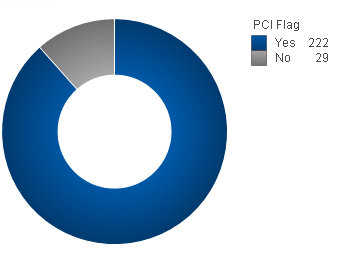# New to QlikView

Discussion board where members can get started with QlikView.

Contributor II

## Calculated Expression

Hi there,

I have been looking around but for some reason am having trouble finding the syntax for what I'm looking for.

I have a dimension "PCI Flag" and it can either be flagged "Yes" or "No".  I have a doughnut chart just showing the two fields and as "Text in Chart" I would like to have a calculated expression showing the % of "Yes".So, I know this is absolutely terrible syntax, but the calculation I want is something like '=(PCI Flag = Yes) / ((PCI Flag = Yes) + (PCI Flag = No))'

I know I need to use something more like  ( {\$<[PCI Flag]={Yes}>} [PCI Flag]) but I am just unsure of the exact syntax that I need.

Any help would be greatly appreciated!

1 Solution

Accepted Solutions
Contributor II

## Re: Calculated Expression

Thanks for all the help everyone!  I actually got it all figured out.

=num(count({<[PCI Flag] = {'Yes'}>}[Field ID]) / count({<[PCI Flag] = {'Yes', 'No'}>}[Field ID]), '##.##%')

7 Replies
Valued Contributor

## Re: Calculated Expression

Use

Sum({<[PCI Flag]={'Yes'}>} Amt)/(Sum({<[PCI Flag]={'Yes'}>} Amt)+Sum({<[PCI Flag]={'No'}>} Amt))

or

Sum({<[PCI Flag]={'Yes'}>} Amt)/(Sum(Amt)

Regards

PratyushPartner

## Re: Calculated Expression

Do you need to provide the % of Yes and not for NoPartner

## Re: Calculated Expression

Hope attached sample will help if you need to show the % of both No & Yes

Contributor II

## Re: Calculated Expression

Yeah just the % of yes.  So in the picture it's Yes = 222; No = 29.  What I want to show is 222/(222+29), which would be 88.45%Partner

## Re: Calculated Expression

Esteemed Contributor II

## Re: Calculated Expression

Dimension

[PCI Flag]

Expression

=count([PCI Flag]) / count(TOTAL [PCI Flag])

Contributor II

## Re: Calculated Expression

Thanks for all the help everyone!  I actually got it all figured out.

=num(count({<[PCI Flag] = {'Yes'}>}[Field ID]) / count({<[PCI Flag] = {'Yes', 'No'}>}[Field ID]), '##.##%')